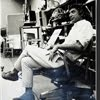## Eugene Ray SDSU 1970'sEugene Ray 1970's. photo credit: Tom Davis

## Thursday, January 30, 2014

### SITCHIN-VON DANIKEN-FLANIGAN STARS / of our scholarship

+SYNCHRONICITY+
>was a popular glue that held our memoir<
>together recorded at SDSU yesterday by<
+DR. SUSAN RESNIK+
>for professor robert ray of the impressive<
+SDSU LIBRARY ARCHIVE+
^^^^^^^^^^^^^^^^^^^^
>i was overwhelmed being back on the<
>campus i hardly recognized with all<
+GRATEFUL FOR THE+
+HONOR TO ME+ !
>its large diverse urbanized buildings<
^^^^^^^^^^^^^^^^^^^^
>eric von daniiken, (above) , zecharia sitchin and<
>dr. patrick flanigan were brought to my mind<
+BIOTRONIC ENERGY+
>as the recording was made where we built<
>pyramids on the campus decades ago<
^^^^^^^^^^^^^^^^^^^^^^^^^
>pyramid power, as dr. flanigan called it, is<
>very much in the news with large testing<
+BIOTRONIC ENERGY+
>structures built in russia in recent times<
^^^^^^^^^^^^^^^^^^^^^^^^^^^^^
>wun chok bong's research and impressive<
>book plus the ancient alien tv programs<
+THE GODS MACHINES+
+ANCIENT EGYPT+
>have supported our own research<
>at SDSU over many years<
^^^^^^^^^^^^^^^^^^
>master machinist christopher dunn has made<
>a profound effort in proving the amazing<
+BIOTRONIC ENERGY+
>accomplishment of the egyptian ET<
>alliance in their pyramid structures<
^^^^^^^^^^^^^^^^^^^^^^^^>my own designs using biotronic energy<
>incorporated conoids (pyramid energy)<
>in public health oriented installation<
^^^^^^^^^^^^^^^^^^^^^^^^^^
>i developed a collage rendering technique to<
>express the radiance of the biotronic design<
>(flouresent surface under ultraviolet light)<
^^^^^^^^^^^^^^^^^^^^^^^^^^^
>later i designed several prototypes using<
>the native americans in san diego and<
>a freeway gate over highway five<
^^^^^^^^^^^^^^^^^^^^^^^

+MODERN RUSSIAN PYRAMID+
+RESEARCH & TESTING+
>is very impressive<
^^^^^^^^^^^^
>dr. alexander golod, phd, has been building<
>and testing over twenty large pyramids in<
>8 different russia and ukraine locations<
^^^^^^^^^^^^^^^^^^^^^^^^^^^>this, the largest of the fiberglass pyramids<
>is 144 feet high and weighs over 55 tons<
>(russia is investing millions of dollars)<
^^^^^^^^^^^^^^^^^^^^^^^
>many different testings are being done<
>including studies in medicine, ecology<
>agriculture, physics & health science<
^^^^^^^^^^^^^^^^^^^^^^^^^
+HUMANS & PLANTS+
>benefitting from experience<
^^^^^^^^^^^^^^^^^^
>"modern russian pyramids"<
>for the impressive results<
^^^^^^^^^^^^^^^^^^
>the radiance from pyramids is effective<
>outside<as well as inside the pyramids<
^^^^^^^^^^^^^^^^^^^^^^^^^^
>recent extensive programming on ancient<
+ANCIENT ALIENS ON+
+HISTORY CHANNEL+
>pyramids in egypt, china and bosnia have<
>suggested the potential of pyramid energy<
^^^^^^^^^^^^^^^^^^^^^^^^^^^^
+PROJECTED INTO SPACE+
+EVEN INTO DEEP SPACE+ !
^^^^^^^^^^^^^^^^^^^
eugene ray, mfa, architect
professor emeritus, sdsu